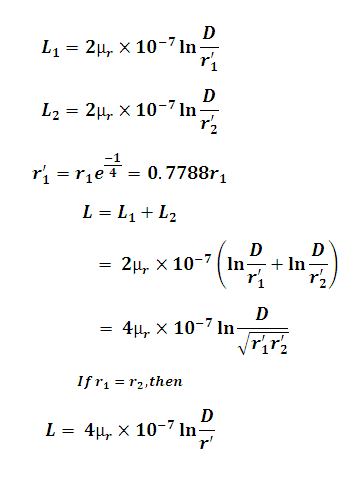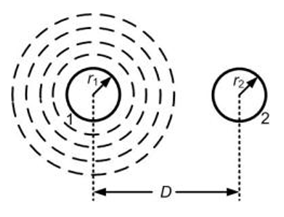# Inductance of Single Phase Transmission Line Calculator

This Calctown Calculator calculates the inductance of a single phase transmission line.

Here µ0 = 4p * 10-7. Please enter the RELATIVE permeability accordingly.

m
m
m
unit

#### Result

H
H
HClick here to view image

where

L1, L2 are individual line inductance

D = distance between the lines

r1, r2 are individual line radii

r' = effective radius

μr = relative permeability